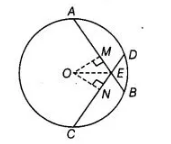# If two equal chords of a circle intersect,`
Question:

If two equal chords of a circle intersect, prove that the parts of one chord are separately equal to the parts of the other chord.

Solution:

Given Consider $A B$ and $C D$ are two equal chords of a circle, which meet at point $E$.

To prove $A E=C E$ and $B E=D E$

Construction Draw $O M \perp A B$ and $O N \perp C D$ and join $O E$ where $O$ is the centre of circle.Proof in $\triangle O M E$ and $\triangle O N E$,

$O M=O N$ [equal chords are equidistant from the centre]

$O E=O E \quad$ [common side]

and $\quad \angle O M E=\angle O N E \quad$ [each 90°]

$\begin{array}{lll}\therefore & \Delta O M E \cong \Delta O N E & \text { [by RHS congurence rule] }\end{array}$

$\Rightarrow \quad E M=E N \quad$ [by CPCT]...(i)

Now, $\quad A B=C D$

On dividing both sides by 2 , we get

$\frac{A B}{2}=\frac{C D}{2} \Rightarrow A M=C N$ ...(ii)

[since, perpendicular drawn from centre of circle to chord bisects the chord $i . e ., A M=M B$ and $C N=N D$ ]

On adding Eqs. (i) and (ii), we get

$E M+A M=E N+C N$

$\Rightarrow \quad A E=C E \quad$... (iii)

Now, $A B=C D$

On subtracting both sides by $A E$, we get

$A B-A E=C D-A E$

$\Rightarrow \quad B E=C D-C E \quad$ [from Eq. (iii)]

$\Rightarrow \quad B E=D E$

Hence proved.